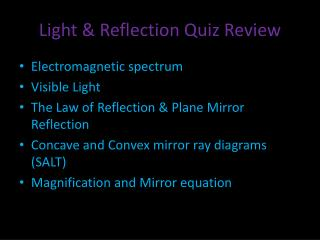DownloadDownload PresentationLight & Reflection Quiz Review

# Light & Reflection Quiz Review

Download Presentation## Light & Reflection Quiz Review

- - - - - - - - - - - - - - - - - - - - - - - - - - - E N D - - - - - - - - - - - - - - - - - - - - - - - - - - -
##### Presentation Transcript

1. Light & Reflection Quiz Review • Electromagnetic spectrum • Visible Light • The Law of Reflection & Plane Mirror Reflection • Concave and Convex mirror ray diagrams (SALT) • Magnification and Mirror equation

2. Electromagnetic Spectrum

3. Visible Light • Incandescent Light • Fluorescent • Phosphorescent Light • Chemiluminescence-Bioluminescence • Triboluminescence • Electric Charge • LED (OLED) • Plasma Displays • Liquid Crystal Displays

4. Law of Reflection

5. Plane Mirror Reflection

6. Concave & Convex Mirror Diagrams • Concave and convex mirror uses • Real vs. Virtual images • Ray Diagrams: • Behaviour of light when it hits a curved mirror • Vertex, Principal Axis, Center of Curvature, Focal Point, Focal length, Normal • Characteristics and positions of images (SALT)

7. Concave vs. Convex mirrors When rays that are parallel to the principal axis strike a concave mirror, they are ALL reflected through the same point, called the focal point. If several groups of parallel rays are reflected by a concave mirror, each group converges at a point.

8. Concave vs. Convex mirrors • Concave mirrors are also called converging mirrors • Concave mirrors are designed to collect light and bring it to a single point • Examples: Cosmetic mirrors to produce an enlarged image, Telescopes to collect light from a distance source and focus it for viewing • Concave mirrors can also be used to send out beams of light rays • Examples: flashlights, car headlights, dental examination lights

9. Concave vs. Convex mirrors

10. Concave vs. Convex mirrors • Convex mirrors are also called diverging mirrors • Convex mirrors spread out rays • They allow you to view a large region that you could not see with a plane mirror of the same size • Examples: Store security, parking lot safety, side-view mirrors, camera phones

11. Real vs. Virtual • A real image is formed by light rays that converge at the location of the image. • A real image can be projected onto a screen: If you place a piece of paper at the spot where a real image forms, a focused image will appear on the piece of paper. • A virtual image is any image formed by rays that do not actually pass through the location of the image. • Light rays are not coming from where the image appears to be. • Unlike a real image, a virtual image cannot be projected onto a screen.

12. Curved mirror definitions • When parallel light rays strike a curved mirror, the reflected rays eventually meet at a common point. The point where the light rays meet, or appear to meet, is called the focal point, F. • The middle of a curved mirror is the vertex. The principal axisis an imaginary line draw through the vertex. • The distance from the vertex to the focal point is the focal length, f. • The center of curvature, C, is the center of the circle that would be formed if you extended the curve of the mirror. The focal point is half way between the center of curvature and the vertex.

13. Finding the image Each time you find the image, you must determine the following: • Size: Is it smaller, bigger, or the same size as the object? • Attitude: Is it upright or inverted? • Location: Is it behind or in front of the mirror? • Type: Is it real of virtual?

14. Equations • Magnification +/- M • Mirror Equation • Positive focal length for concave mirrors • Negative focal length for convex mirrors ***SIGN CONVENTIONS